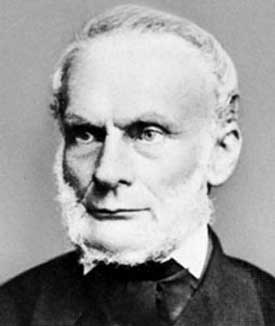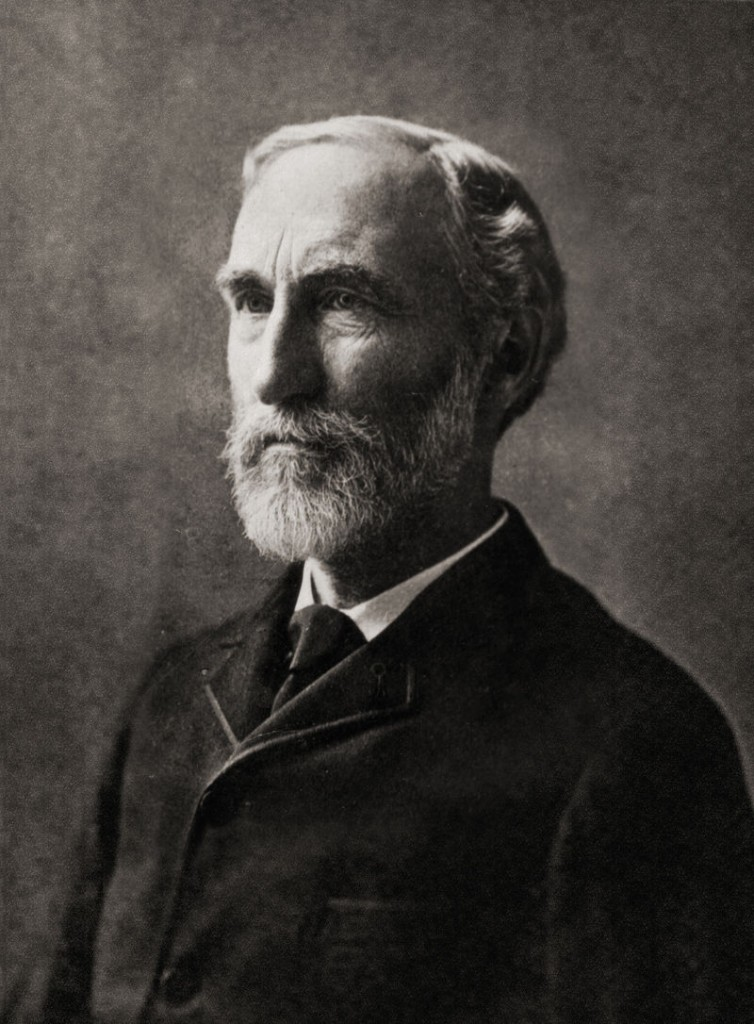# Difference Between Entropy and Enthalpy

## Main Difference – Entropy vs Enthalpy

Both Entropy and Enthalpy relate to functions of measurements in chemical thermodynamics. And also they are both related to the heat changes of a reaction. The main difference between entropy and enthalpy is, entropy is used as a measurement of the disorder or the randomness of a chemical process while enthalpy is used as a measure of the heat change of a chemical reaction or the change in internal energy of a reaction under constant pressure.

## What is Entropy

As mentioned above, entropy measures the randomness or the extent of disorder in a chemical process. According to the second law of thermodynamics, it is believed that the entropy of an isolated system will always increase. What this means is that chemical reactions will tend to drive themselves in the direction of more disorder. This is when a process becomes stable. The randomness or the disorder of a reaction increases as the number of molecules increases. Therefore, if a reaction has a higher number of molecules as products than its reactants, it can be concluded that the reaction is moving towards a higher level of disorder, and this is a favourable condition in chemistry.

Entropy is identified as a state function in chemistry as the change in entropy doesn’t depend on the chemical path travelled when moving from the chemical reactants to the products. It only depends on the beginning and the ending points of a reaction. Entropy is symbolized by ‘S’, and as it is a state function, it is always written as a capital letter. A change in entropy is written as ‘ΔS’. Entropy can be mathematically expressed as the division between the change in heat and temperature. However, chemical processes can be either reversible or irreversible. And the heat change during the reversible process is what is taken into account when deriving the equations for entropy as it involves the maximum heat transfer. The total change in entropy during a chemical reaction is the difference between the entropy of the products and the entropy of the reactants. Entropy can be measured in units JK-1.Rudolf Clausius is the originator of the concept of entropy

## What is Enthalpy

The change in enthalpy relates to the heat change of a reaction, and when the reaction occurs at constant pressure, it represents the internal energy change of the reaction system. It makes little sense to measure the enthalpy directly, whereas the change in enthalpy is what carries meaning. Enthalpy is also a state function which means the value will not change depending on the chemical route taken to obtain the products. As it is a state function, it is denoted by a capital letter and, in this case, it is ‘H’, and a change in enthalpy is marked as ‘ΔH’. The total change in enthalpy of a reaction is the difference between the enthalpy of the products and the enthalpy of the reactants. Enthalpy is measured in the units of Jmol-1.

The heat change of a reaction is referred to as its enthalpy only when it takes place under standard conditions. That is at a pressure of 1 bar and a designated temperature which is generally 25°C. Many types of reaction enthalpies exist. i.e. Enthalpy of reaction, enthalpy of formation, enthalpy of combustion, enthalpy of neutralization, enthalpy of solution, etc.Josiah Willard Gibbs’ theories were the earliest writings to contain the concept of enthalpy.

## Difference Between Entropy and Enthalpy

### Definition

Entropy is a measure of the randomness or the extent of disorder of a chemical process.

Enthalpy is a measure of the heat change of a reaction occurring at a constant pressure.

### Measurement Units

Entropy is measured in JK-1

Enthalpy is measured in Jmol-1

### Requirements

Entropy has no requirements or limits, and its change is measured by the division between the heat change of the chemical process and the temperature.

In contrast, enthalpy can only be related to the heat change of the reaction under standard conditions.

Image Courtesy:

“Clausius” by Original uploader was user: Sadi Carnot – (Public Domain) via Commons

“Josiah Willard Gibbs -from MMS-” by Unknown. Uploaded by Serge Lachinov (обработка для wiki) – Frontispiece of The Scientific Papers of J. Willard Gibbs, in two volumes, eds. H. A. Bumstead and R. G. Van Name, (London and New York: Longmans, Green, and Co., 1906). (Public Domain) via Commons